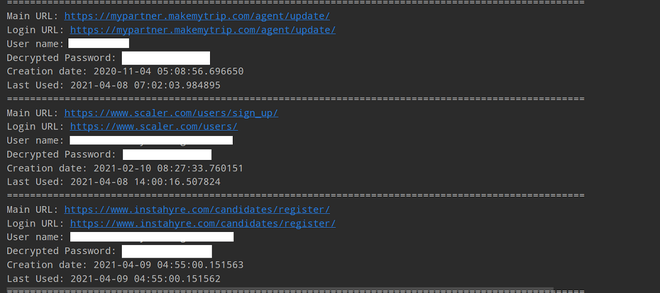Related Articles

# How to Extract Chrome Passwords in Python?

• Last Updated : 21 Apr, 2021

Note: This article is for users who use Chrome on Windows. If you are a Mac or Linux user, you may need to make some changes to the given path, while the rest of the Python program will remain the same.

### Installation:

Now, Let’s install some important libraries which we need to write a python program through which we can extract Chrome Passwords.

```pip install pycryptodome
pip install pypiwin32```

Before we extract the password directly from Chrome, we need to define some useful functions that will help our main functions.

• First Function
```def chrome_date_and_time(chrome_data):

# Chrome_data format is
# year-month-date hr:mins:seconds.milliseconds
# This will return datetime.datetime Object
return datetime(1601, 1, 1) + timedelta(microseconds=chrome_data)```

The chrome_date_and_time() function is responsible for converting Chrome’s date format into a human-readable date and time format.

Chrome Date and time format look like this:

`'year-month-date hr:mins:seconds.milliseconds'`

Example:

`2020-06-01 10:49:01.824691`
• Second Function
```def fetching_encryption_key():

# Local_computer_directory_path will
# look like this below
# C: => Users => <Your_Name> => AppData =>
# Local => Google => Chrome => User Data =>
# Local State

local_computer_directory_path = os.path.join(
"Chrome", "User Data", "Local State")

with open(local_computer_directory_path, "r", encoding="utf-8") as f:

# decoding the encryption key using base64
encryption_key = base64.b64decode(
local_state_data["os_crypt"]["encrypted_key"])

# remove Windows Data Protection API (DPAPI) str
encryption_key = encryption_key[5:]

# return decrypted key
return win32crypt.CryptUnprotectData(
encryption_key, None, None, None, 0)```

The fetching_encryption_key() function obtains and decodes the AES key used to encrypt the password. It is saved as a JSON file in “C:\Users\<Your_PC_Name>\AppData\Local\Google\Chrome\User Data\Local State”. This function will be useful for the encrypted key.

• Third Function
```def password_decryption(password, encryption_key):

try:

# generate cipher
cipher = AES.new(encryption_key, AES.MODE_GCM, iv)

except:
try:
return str(win32crypt.CryptUnprotectData(password, None, None, None, 0))
except:

Below is the implementation.

## Python3

 `import` `os ` `import` `json ` `import` `base64 ` `import` `sqlite3 ` `import` `win32crypt ` `from` `Cryptodome.Cipher ``import` `AES ` `import` `shutil ` `from` `datetime ``import` `timezone, datetime, timedelta ` ` `  ` `  `def` `chrome_date_and_time(chrome_data): ` `    ``# Chrome_data format is 'year-month-date  ` `    ``# hr:mins:seconds.milliseconds ` `    ``# This will return datetime.datetime Object ` `    ``return` `datetime(``1601``, ``1``, ``1``) ``+` `timedelta(microseconds``=``chrome_data) ` ` `  ` `  `def` `fetching_encryption_key(): ` `    ``# Local_computer_directory_path will look  ` `    ``# like this below ` `    ``# C: => Users => => AppData => ` `    ``# Local => Google => Chrome => User Data => ` `    ``# Local State ` `    ``local_computer_directory_path ``=` `os.path.join( ` `      ``os.environ[``"USERPROFILE"``], ``"AppData"``, ``"Local"``, ``"Google"``, ``"Chrome"``,  ` `      ``"User Data"``, ``"Local State"``) ` `     `  `    ``with ``open``(local_computer_directory_path, ``"r"``, encoding``=``"utf-8"``) as f: ` `        ``local_state_data ``=` `f.read() ` `        ``local_state_data ``=` `json.loads(local_state_data) ` ` `  `    ``# decoding the encryption key using base64 ` `    ``encryption_key ``=` `base64.b64decode( ` `      ``local_state_data[``"os_crypt"``][``"encrypted_key"``]) ` `     `  `    ``# remove Windows Data Protection API (DPAPI) str ` `    ``encryption_key ``=` `encryption_key[``5``:] ` `     `  `    ``# return decrypted key ` `    ``return` `win32crypt.CryptUnprotectData(encryption_key, ``None``, ``None``, ``None``, ``0``)[``1``] ` ` `  ` `  `def` `password_decryption(password, encryption_key): ` `    ``try``: ` `        ``iv ``=` `password[``3``:``15``] ` `        ``password ``=` `password[``15``:] ` `         `  `        ``# generate cipher ` `        ``cipher ``=` `AES.new(encryption_key, AES.MODE_GCM, iv) ` `         `  `        ``# decrypt password ` `        ``return` `cipher.decrypt(password)[:``-``16``].decode() ` `    ``except``: ` `         `  `        ``try``: ` `            ``return` `str``(win32crypt.CryptUnprotectData(password, ``None``, ``None``, ``None``, ``0``)[``1``]) ` `        ``except``: ` `            ``return` `"No Passwords"` ` `  ` `  `def` `main(): ` `    ``key ``=` `fetching_encryption_key() ` `    ``db_path ``=` `os.path.join(os.environ[``"USERPROFILE"``], ``"AppData"``, ``"Local"``, ` `                           ``"Google"``, ``"Chrome"``, ``"User Data"``, ``"default"``, ``"Login Data"``) ` `    ``filename ``=` `"ChromePasswords.db"` `    ``shutil.copyfile(db_path, filename) ` `     `  `    ``# connecting to the database ` `    ``db ``=` `sqlite3.connect(filename) ` `    ``cursor ``=` `db.cursor() ` `     `  `    ``# 'logins' table has the data ` `    ``cursor.execute( ` `        ``"select origin_url, action_url, username_value, password_value, date_created, date_last_used from logins "` `        ``"order by date_last_used"``) ` `     `  `    ``# iterate over all rows ` `    ``for` `row ``in` `cursor.fetchall(): ` `        ``main_url ``=` `row[``0``] ` `        ``login_page_url ``=` `row[``1``] ` `        ``user_name ``=` `row[``2``] ` `        ``decrypted_password ``=` `password_decryption(row[``3``], key) ` `        ``date_of_creation ``=` `row[``4``] ` `        ``last_usuage ``=` `row[``5``] ` `         `  `        ``if` `user_name ``or` `decrypted_password: ` `            ``print``(f``"Main URL: {main_url}"``) ` `            ``print``(f``"Login URL: {login_page_url}"``) ` `            ``print``(f``"User name: {user_name}"``) ` `            ``print``(f``"Decrypted Password: {decrypted_password}"``) ` `         `  `        ``else``: ` `            ``continue` `         `  `        ``if` `date_of_creation !``=` `86400000000` `and` `date_of_creation: ` `            ``print``(f``"Creation date: {str(chrome_date_and_time(date_of_creation))}"``) ` `         `  `        ``if` `last_usuage !``=` `86400000000` `and` `last_usuage: ` `            ``print``(f``"Last Used: {str(chrome_date_and_time(last_usuage))}"``) ` `        ``print``(``"="` `*` `100``) ` `    ``cursor.close() ` `    ``db.close() ` `     `  `    ``try``: ` `         `  `        ``# trying to remove the copied db file as  ` `        ``# well from local computer ` `        ``os.remove(filename) ` `    ``except``: ` `        ``pass` ` `  ` `  `if` `__name__ ``=``=` `"__main__"``: ` `    ``main() `

Output:For the above code, we followed these below steps;

• First, we use the previously defined function fetching_encryption_key() to obtain the encryption key
• Then copy the SQLite database in “C:\Users\<Your_PC_Name>\AppData\Local\Google\Chrome\User Data\default\Login Data” where the saved Password data is stored of the current directory and establish a connection with it. This is because the original database file locked when Chrome started.
• With the help of the cursor object, we will execute the SELECT SQL query from the ‘logins’ table order by date_last_used.
• Traverse all the login rows in a more readable format to obtain the passwords for each password and format date_created and date_last_used.
• Finally, With the help of print statements, we will print all the saved credentials which are extracted from Chrome.
• Delete the copy of the database from the current directory.

Attention geek! Strengthen your foundations with the Python Programming Foundation Course and learn the basics.

To begin with, your interview preparations Enhance your Data Structures concepts with the Python DS Course. And to begin with your Machine Learning Journey, join the Machine Learning – Basic Level Course

My Personal Notes arrow_drop_up
Recommended Articles
Page :